# RD Sharma Solutions for Class 11 Chapter 17 - Combinations Exercise 17.3

We shall discuss some practical problems where both permutations and combinations are used in a single problem. The explanation provided in the solution pdf enhances the basic concepts among the students, which in turn helps in solving problems without any confusion. Students can also assess their knowledge gap with the help of these solutions and study accordingly. Students are also advised to practice these solutions on a regular basis, which helps in yielding good marks in their exams. RD Sharma Class 11 Maths Solutions are available here in a simple pdf format, which can be downloaded easily from the given links.

## Download the pdf of RD Sharma Solutions for Class 11 Maths Exercise 17.3 Chapter 17 – Combinations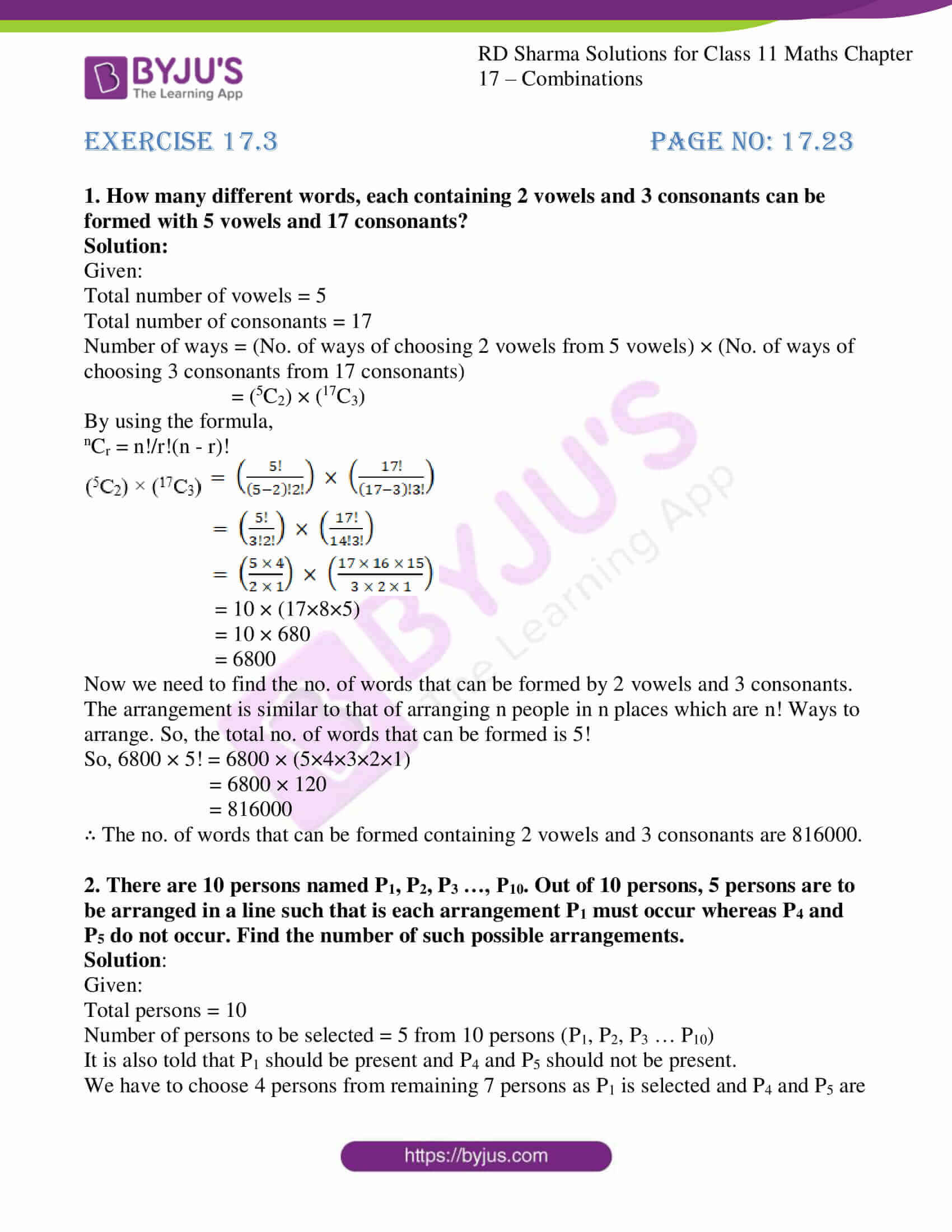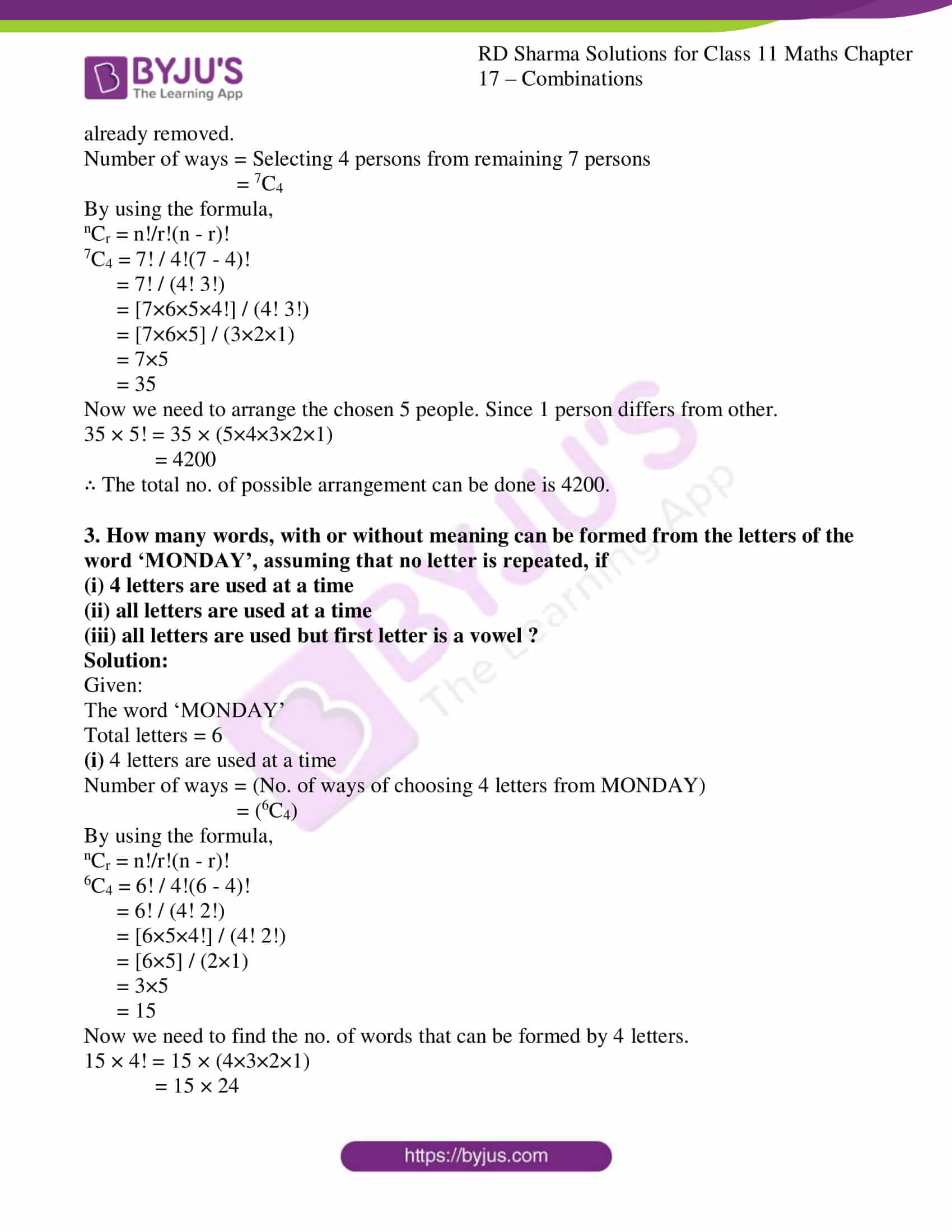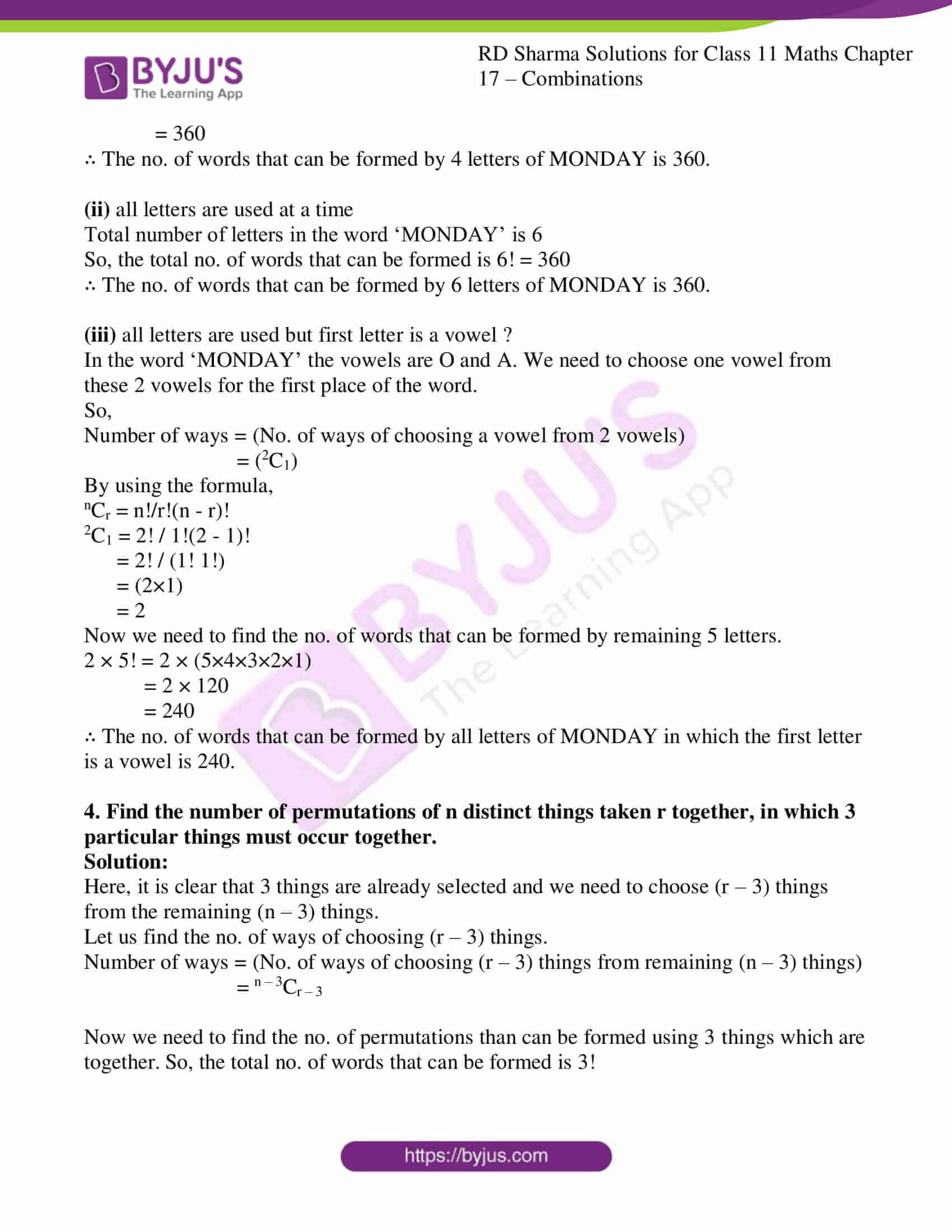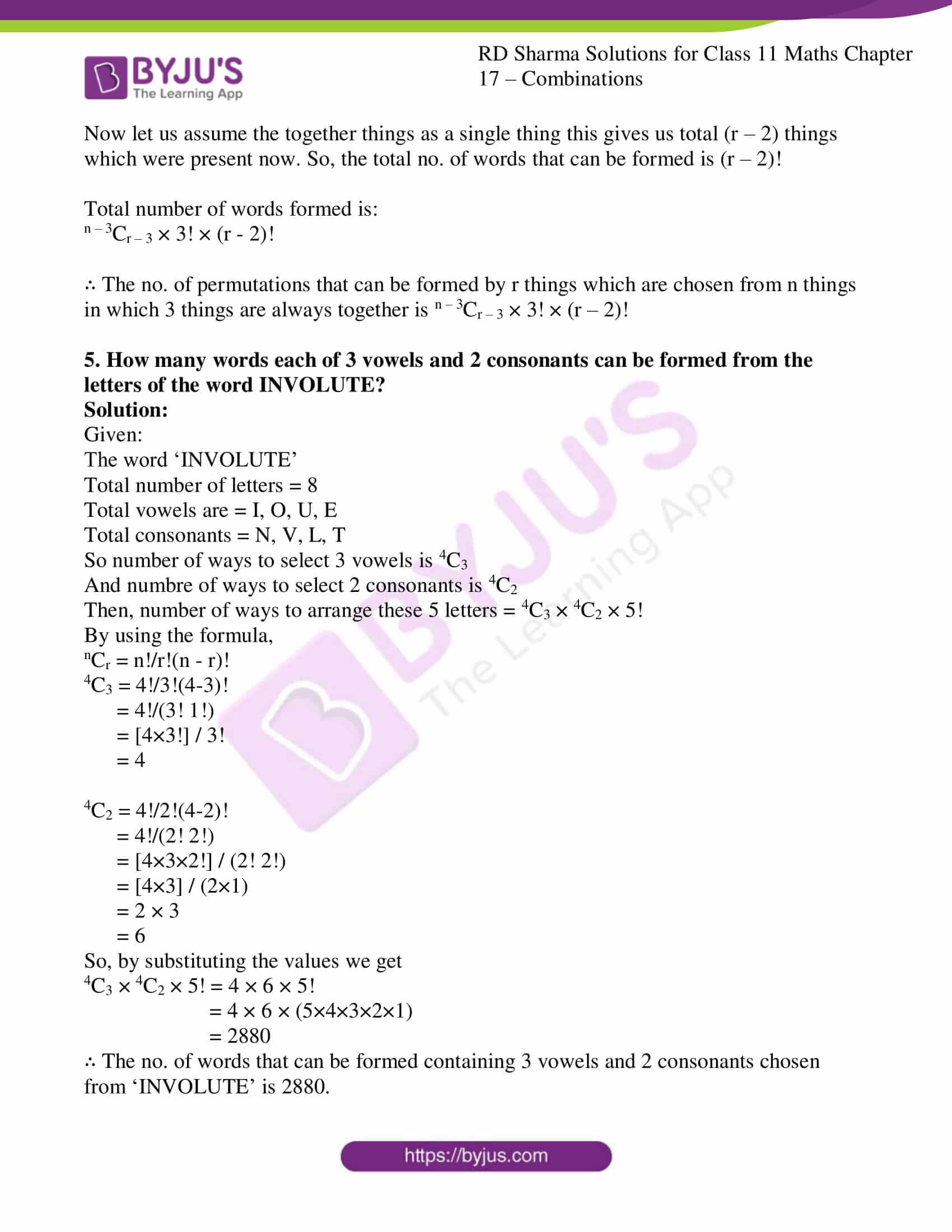### Access answers to RD Sharma Solutions for Class 11 Maths Exercise 17.3 Chapter 17 – Combinations

1. How many different words, each containing 2 vowels and 3 consonants can be formed with 5 vowels and 17 consonants?

Solution:

Given:

Total number of vowels = 5

Total number of consonants = 17

Number of ways = (No. of ways of choosing 2 vowels from 5 vowels) × (No. of ways of choosing 3 consonants from 17 consonants)

= (5C2) × (17C3)

By using the formula,

nCr = n!/r!(n – r)!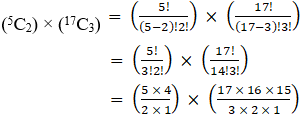= 10 × (17×8×5)

= 10 × 680

= 6800

Now we need to find the no. of words that can be formed by 2 vowels and 3 consonants.

The arrangement is similar to that of arranging n people in n places which are n! Ways to arrange. So, the total no. of words that can be formed is 5!

So, 6800 × 5! = 6800 × (5×4×3×2×1)

= 6800 × 120

= 816000

∴ The no. of words that can be formed containing 2 vowels and 3 consonants are 816000.

2. There are 10 persons named P1, P2, P3 …, P10. Out of 10 persons, 5 persons are to be arranged in a line such that is each arrangement P1 must occur whereas P4 and P5 do not occur. Find the number of such possible arrangements.

Solution:

Given:

Total persons = 10

Number of persons to be selected = 5 from 10 persons (P1, P2, P3 … P10)

It is also told that P1 should be present and P4 and P5 should not be present.

We have to choose 4 persons from remaining 7 persons as P1 is selected and P4 and P5 are already removed.

Number of ways = Selecting 4 persons from remaining 7 persons

7C4

By using the formula,

nCr = n!/r!(n – r)!

7C4 = 7! / 4!(7 – 4)!

= 7! / (4! 3!)

= [7×6×5×4!] / (4! 3!)

= [7×6×5] / (3×2×1)

= 7×5

= 35

Now we need to arrange the chosen 5 people. Since 1 person differs from other.

35 × 5! = 35 × (5×4×3×2×1)

= 4200

∴ The total no. of possible arrangement can be done is 4200.

3. How many words, with or without meaning can be formed from the letters of the word ‘MONDAY’, assuming that no letter is repeated, if
(i) 4 letters are used at a time

(ii) all letters are used at a time

(iii) all letters are used but first letter is a vowel ?

Solution:

Given:

The word ‘MONDAY’

Total letters = 6

(i) 4 letters are used at a time

Number of ways = (No. of ways of choosing 4 letters from MONDAY)

= (6C4)

By using the formula,

nCr = n!/r!(n – r)!

6C4 = 6! / 4!(6 – 4)!

= 6! / (4! 2!)

= [6×5×4!] / (4! 2!)

= [6×5] / (2×1)

= 3×5

= 15

Now we need to find the no. of words that can be formed by 4 letters.

15 × 4! = 15 × (4×3×2×1)

= 15 × 24

= 360

∴ The no. of words that can be formed by 4 letters of MONDAY is 360.

(ii) all letters are used at a time

Total number of letters in the word ‘MONDAY’ is 6

So, the total no. of words that can be formed is 6! = 360

∴ The no. of words that can be formed by 6 letters of MONDAY is 360.

(iii) all letters are used but first letter is a vowel ?

In the word ‘MONDAY’ the vowels are O and A. We need to choose one vowel from these 2 vowels for the first place of the word.

So,

Number of ways = (No. of ways of choosing a vowel from 2 vowels)

= (2C1)

By using the formula,

nCr = n!/r!(n – r)!

2C1 = 2! / 1!(2 – 1)!

= 2! / (1! 1!)

= (2×1)

= 2

Now we need to find the no. of words that can be formed by remaining 5 letters.

2 × 5! = 2 × (5×4×3×2×1)

= 2 × 120

= 240

∴ The no. of words that can be formed by all letters of MONDAY in which the first letter is a vowel is 240.

4. Find the number of permutations of n distinct things taken r together, in which 3 particular things must occur together.

Solution:

Here, it is clear that 3 things are already selected and we need to choose (r – 3) things from the remaining (n – 3) things.

Let us find the no. of ways of choosing (r – 3) things.

Number of ways = (No. of ways of choosing (r – 3) things from remaining (n – 3) things)

n – 3Cr – 3

Now we need to find the no. of permutations than can be formed using 3 things which are together. So, the total no. of words that can be formed is 3!

Now let us assume the together things as a single thing this gives us total (r – 2) things which were present now. So, the total no. of words that can be formed is (r – 2)!

Total number of words formed is:

n – 3Cr – 3 × 3! × (r – 2)!

∴ The no. of permutations that can be formed by r things which are chosen from n things in which 3 things are always together is n – 3Cr – 3 × 3! × (r – 2)!

5. How many words each of 3 vowels and 2 consonants can be formed from the letters of the word INVOLUTE?

Solution:

Given:

The word ‘INVOLUTE’

Total number of letters = 8

Total vowels are = I, O, U, E

Total consonants = N, V, L, T

So number of ways to select 3 vowels is 4C3

And numbre of ways to select 2 consonants is 4C2

Then, number of ways to arrange these 5 letters = 4C3 × 4C2 × 5!

By using the formula,

nCr = n!/r!(n – r)!

4C3 = 4!/3!(4-3)!

= 4!/(3! 1!)

= [4×3!] / 3!

= 4

4C2 = 4!/2!(4-2)!

= 4!/(2! 2!)

= [4×3×2!] / (2! 2!)

= [4×3] / (2×1)

= 2 × 3

= 6

So, by substituting the values we get

4C3 × 4C2 × 5! = 4 × 6 × 5!

= 4 × 6 × (5×4×3×2×1)

= 2880

∴ The no. of words that can be formed containing 3 vowels and 2 consonants chosen from ‘INVOLUTE’ is 2880.